Next: Lower Oracle Query Bounds Up: Introduction Previous: Lower Bounds   Contents

# Summary

In Chapter 2, we define the quantum oracle model, and present Ambainis' Theorem for proving lower bounds within this framework. We examine a shortcoming of Ambainis' Theorem, and then derive two theorems and use them to prove lower query bounds for symmetric functions.

In Chapter 3, we apply the results of Chapter 2 to the interesting and well studied case of non-trivial monotone graph properties. Finding the results wanting we appeal directly to Ambainis' Theorem to establish new lower bounds for graph connectivity and bipartiteness. Finally we show there is no quantum extension of the Aanderaa-Karp-Rosenberg conjecture in the bounded error setting.

In Chapter 4, we examine classes of functions that do not have the inherent symmetries of those in chapters 2 and 3. We provide lower query bounds for tree functions, nondeterministically evasive functions, and sensitive functions.

We conclude in Chapter 5 with a brief examination of some open questions for lower oracle query bounds in the bounded error setting, and quantum computing in general.

Many of the results in this thesis have been attained previously by other authors. We present these results again to illustrate how Ambainis' Theorem can provide relatively simpler proofs for a variety of problems. Table 1.1 summarizes our results and credits previous papers where appropriate. All results are in the quantum bounded error setting.

Table 1.1: Summary of Results
 Function Lower Query Bound Section Reference Generalized XOR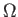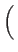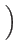2.2.1 Determining the Oracle String N/2 2.3 Singleton functions2.4 Partially symmetric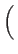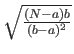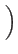2.5 AND2.6 Beals et al.  OR2.6 Beals et al.  MAJORITY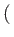N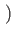2.6 Beals et al.  PARITYN2.6 Beals et al.  Nonconstant symmetric2.7 Beals et al.  Graph connectivityV3.2.1 Graph bipartitenessV3.2.2 Tree functions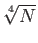4.1 Beals et al.  Nondeterministically evasive4.3 Sensitive functions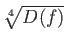4.4Next: Lower Oracle Query Bounds Up: Introduction Previous: Lower Bounds   Contents
Matthew Hayward Lower Query Bounds in the Quantum Oracle Model GitHub Repository